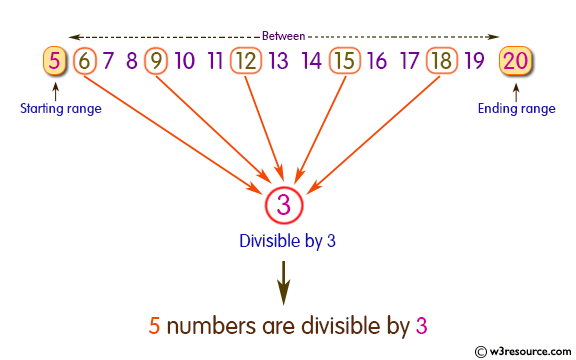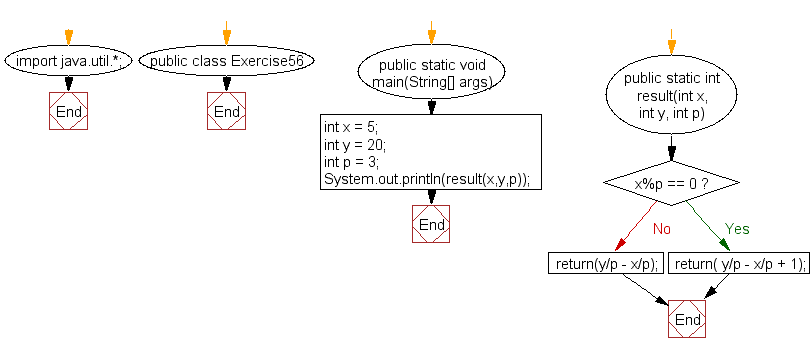﻿ Java: Number of values in a range divisible by a given value# Java Exercises: Find the number of values in a given range divisible by a given value

## Java Basic: Exercise-56 with Solution

Write a Java program to find the number of values in a given range divisible by a given value.
Sample Data:
For example x = 5, y=20 and p =3, find the number of integers within the range x..y and that are divisible by p i.e. { i :x ≤ i ≤ y, i mod p = 0 }

Sample Solution:

Java Code:

``````import java.util.*;
public class Exercise56 {
public static void main(String[] args){
int x = 5;
int y = 20;
int p = 3;
System.out.println(result(x,y,p));
}
public static int result(int x, int y, int p) {
if (x%p == 0)
return( y/p - x/p + 1);
return(y/p - x/p);
}
}
```
```

Sample Output:

```5
```

Pictorial Presentation:Flowchart:Java Code Editor:

What is the difficulty level of this exercise?

Test your Programming skills with w3resource's quiz.

﻿

## Java: Tips of the Day

countOccurrences

Counts the occurrences of a value in an array.

Use Arrays.stream().filter().count() to count total number of values that equals the specified value.

```public static long countOccurrences(int[] numbers, int value) {
return Arrays.stream(numbers)
.filter(number -> number == value)
.count();
}
```

Ref: https://bit.ly/3kCAgLb